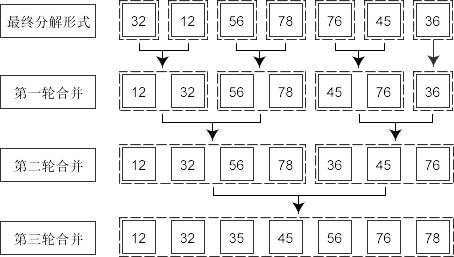# C语言合并排序实例代码

(1)将所要进行的排序序列分为左右两个部分，如果要进行排序的序列的起始元素下标为first，最后一个元素的下标为last，那么左右两部分之间的临界点下标mid=(first+last)/2，这两部分分别是A[first … mid]和A[mid+1 … last]。

(2)将上面所分得的两部分序列继续按照步骤(1)继续进行划分，直到划分的区间长度为1。

(3)将划分结束后的序列进行归并排序，排序方法为对所分的n个子序列进行两两合并，得到n/2或n/2+l个含有两个元素的子序列，再对得到的子序列进行合并，直至得到一个长度为n的有序序列为止。

#include <stdio.h>

#include <stdlib.h>

#define N 7

void merge(int arr[], int low, int mid, int high){

int i, k;

int *tmp = (int *)malloc((high-low+1)*sizeof(int));

//申请空间，使其大小为两个

int left_low = low;

int left_high = mid;

int right_low = mid + 1;

int right_high = high;

for(k=0; left_low<=left_high && right_low<=right_high; k++){ // 比较两个指针所指向的元素

if(arr[left_low]<=arr[right_low]){

tmp[k] = arr[left_low++];

}else{

tmp[k] = arr[right_low++];

}

}

if(left_low <= left_high){ //若第一个序列有剩余，直接复制出来粘到合并序列尾

//memcpy(tmp+k, arr+left_low, (left_high-left_low+l)*sizeof(int));

for(i=left_low;i<=left_high;i++)

tmp[k++] = arr[i];

}

if(right_low <= right_high){

//若第二个序列有剩余，直接复制出来粘到合并序列尾

//memcpy(tmp+k, arr+right_low, (right_high-right_low+1)*sizeof(int));

for(i=right_low; i<=right_high; i++)

tmp[k++] = arr[i];

}

for(i=0; i<high-low+1; i++)

arr[low+i] = tmp[i];

free(tmp);

return;

}

void merge_sort(int arr[], unsigned int first, unsigned int last){

int mid = 0;

if(first<last){

mid = (first+last)/2; /* 注意防止溢出 */

/mid = first/2 + last/2;/

//mid = (first & last) + ((first ^ last) >> 1);

merge_sort(arr, first, mid);

merge_sort(arr, mid+1,last);

merge(arr,first,mid,last);

}

return;

}

int main(){

int i;

int a[N]={32,12,56,78,76,45,36};

printf (“排序前 \n”);

for(i=0;i<N;i++)

printf("%d\t",a[i]);

merge_sort(a,0,N-1); // 排序

printf ("\n 排序后 \n");

for(i=0;i<N;i++)

printf("%d\t",a[i]); printf("\n");

system(“pause”);

return 0;

}

32 12 56 78 76 45 36

12 32 36 45 56 76 78©️2019 CSDN 皮肤主题: 技术黑板 设计师: CSDN官方博客